Pagini:    În jos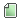Ajutor Subiect: Problem: Shoe laces  (Citit de 3966 ori) 0 Utilizatori şi 1 Vizitator pe acest subiect.
Cosmin
Echipa infoarena
Nu mai tace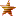Karma: 351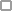Deconectat

Mesaje: 1.799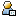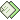« : Aprilie 28, 2016, 07:47:00 »

http://www.infoarena.ro/blog/shoelacesMemorat
harababurel
Client obisnuit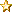Karma: 23Deconectat

Mesaje: 62« Răspunde #1 : Aprilie 28, 2016, 10:29:41 »

I think the following approach might work:

Let dp(i)(j) = the probability  of reaching a state with i untied lace ends and j cycles.

During an operation, two ends are chosen at random and tied together, so they won't be used in the future. This takes us to a state with i-2 untied ends.

Now, there is a 1/(i-1) (or something similar) probability that the two freshly tied ends will close a cycle, and an (i-2)/(i-1) probability that no new cycle will appear.

Therefore we can move to state (i-2)(j+1) with probability P and to (i-2)(j) with probability 1-P.

The trivial state is dp(2*n)(0) = 1.
 « Ultima modificare: Aprilie 28, 2016, 10:36:11 de către Puscas Sergiu »Memorat
devilkind
Echipa infoarena
Nu mai taceKarma: 284Deconectat

Mesaje: 1.240« Răspunde #2 : Aprilie 28, 2016, 12:43:19 »

Not 100% sure but I think this is equivalent with the expected number of cycles in a random permutation of size N.

Also I think the answer is N / 2, not proof of this yet, just a wild guess, because I have a feeling that P(i) = Probability you have i cycles = P(N - i + 1) (in other words the probability of having i cycles is the same as the probability N - i + 1 cycles).Memorat
atatomir
StrainKarma: 3Deconectat

Mesaje: 25« Răspunde #3 : Aprilie 29, 2016, 00:28:05 »

I totally agree with Tiberiu regarding the equivalence between this problem and counting the expected number of cycles in a permutation of n elements , but I have a different formula .
I think that the answer is = 1 + 1/2 + 1/3 + 1/4 + ... + 1/n .
As a proof, I am going to use induction . Let's note E(i) : the expected number of cycles in a permutation of degree i.

E(1) = 1
Let k be given (k > 1), suppose that E(k-1) is true.
Every permutation of n-1 elements can generate other n-1 permutations of n elements (each of them containing the last shoe lace in an already existing cycle)  and one additional permutation where the last shoe lace forms a cycle of length 1. Only the last case increses the expected value ,so we can conclude that E(i) = E(i-1) *  (n-1)/n + ( E(i-1) + 1 ) * 1/n = E(i-1) + 1/n.

I hope my solution is correct and easy to understand .Memorat
Cosmin
Echipa infoarena
Nu mai taceKarma: 351Deconectat

Mesaje: 1.799« Răspunde #4 : Aprilie 29, 2016, 06:10:25 »

FIIAurelian has the right solution.Memorat
 Pagini:    În sus
Schimbă forumul: